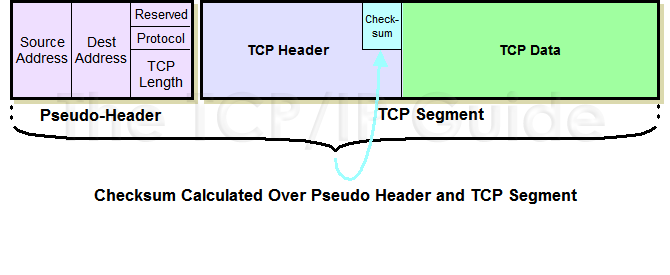# Modify packet [DELPHI]

Home Forums Discussions Support Portal Modify packet [DELPHI]

Viewing 9 posts - 1 through 9 (of 9 total)
• Author
Posts
• #4856

Hi,

I need to modify packet, but how to calculate the new checksum in Delphi

I have tried to translate C++ example, but it doesn’t work 🙁

(sorry for my english).

#5575

Try it. It is universal function.

p – pointer to begin of header.
Size – size of header in words.
Attention: Field Checksum must be = 0 before call function.

function Checksum(p: PWORD; Size: word): WORD;
var
i: Integer;
Sum: DWORD;
begin
Sum := 0;
for i := 0 to Size – 1 do
begin
Sum := Sum + ntohs(p^);
inc(p);
end;
while (Sum shr 16) > 0 do
Sum := (Sum and \$FFFF) + (Sum shr 16);
Sum := not Sum;
Result := Sum;
end;

Examples:
1. Calc checksum for IPHeader. For uses without IPOptions.

(sizeof(ICMP_HDR)+Length(Data)) div 2));

Don`t forget call htons for result of function Checksum.
Sorry for my English.

#5576

But how to calculate TCP checksum ?

I’ve tried your function but the checksum is incorrect

Thanks

#5577

Checksum = calculate (pointer, (header+data) div 2), as for ICMP as TCP

Example:

``````
Packet = ETH_HDR+IP_HDR+TCP_HDR+Data;
IP_HDR.Checksum := 00;
IP_HDR.Checksum := htons(Checksum(Pword(IP_HDR), sizeof(IP_HDR) div 2));

TCP.Checksum := 00;
TCP_HDR.Checksum := htons(Checksum(Pword(TCP_HDR), sizeof((TCP_HDR+Length(Data)) div 2));
``````

For more: See ICMP example in previous reply

#5578

first, i decare this

``````
VerLen: Byte;   //HL
TOS: Byte;
TotalLen: Word;
Identifer: Word;
FragOffsets: Word;
TTL: Byte;
Protocol: Byte;
CheckSum: Word;
SourceIp: DWORD;
DestIp: DWORD;
// Options: DWORD;
end;

SourcePort:Word;
DestPort:Word;
SequenceNumber:DWord;
AcknowledgementNumber:DWord;
Offset:Byte; //only left 4 bits. Header length in 32-bit segments
Flags:Byte;
Window:Word;
UrgentPointer:Word;
end;

pTCPData: PChar;
``````

after that i get IP header and calc IP check sum is collect but why i can’t calc TCP checksum. the code like below.

``````
``````

i forgot something? or i should not use PChar for get the TCP data?

#5579

@PLY wrote:

first, i declare this

``````
VerLen: Byte;   //HL
TOS: Byte;
TotalLen: Word;
Identifer: Word;
FragOffsets: Word;
TTL: Byte;
Protocol: Byte;
CheckSum: Word;
SourceIp: DWORD;
DestIp: DWORD;
// Options: DWORD;
end;

SourcePort:Word;
DestPort:Word;
SequenceNumber:DWord;
AcknowledgementNumber:DWord;
Offset:Byte; //only left 4 bits. Header length in 32-bit segments
Flags:Byte;
Window:Word;
UrgentPointer:Word;
end;

pTCPData: PChar;
``````

after that i get IP header and calc IP check sum is collect but why i can’t calc TCP checksum. the code like below.

``````
``````

i forgot something? or i should not use PChar for get the TCP data?

#5580

I not absolutely understand a question, therefore I shall answer how I have understood it.

``````
...
var
Buffer: INTERMEDIATE_BUFFER;
pData: Pbyte;
DataSize: Integer;
...

const
HSIZE = sizeof(ETHERNET_HDR) + sizeof(IP_HDR)+ sizeof(ICMP_HDR);

...

...

begin
...

// or so
...

// Change Port Destination to 4032

// Tne IPHeader checksum it is not recalculated therefore as the size of a package has not changed

//If the size of a packet has changed, it is necessary to align a packet to word and recalc IP Header and accordingly TCP Header
...

end;
...

``````

In a code there can be mistakes, for what beforehand apologize.

#5581

Hi
i write a program with delphi
but i have problem Correct Calculate TCP CheckSum
i used code write by “heilong”
give correct on IPcheksum And ICMPcheksum
but on UDP & TCP incorect result
i think this code need “pseudo header”
See Picture :and read all fucntion in C++ to calculatechecksum

i can convert this code to DLL and export fucntion:
http://www.netfor2.com/tcpsum.htm
but I do not know used parammerter in delphi

But i can’t wirte a correct algoritm in delphi 🙁
or give me any solution in DELPHI to i can correct TCP & UDP checksum Calculate
.

Edited @00:14 AM , used 🙂 OKY 😯
دهنمو گاید ، ولی بلاخره شد ، ای مردشور هرچی پوینتر هست ببرن 🙂

#5582
``````
var
i,sum: ulong;
begin
sum := 0;
if (aLen div 2) * 2 <> aLen then begin
buff[aLen] := 0;
end;
i := 0;
while i < aLen+padd do begin
w16 := ((buff shl 8)and \$FF00) + (buff[i+1]and \$FF);
sum := sum + dword(w16);
i := i + 2;
end;

sum := sum + ntohs(sIP.S_un_w.s_w1) + ntohs(sIP.S_un_w.s_w2);
sum := sum + ntohs(dIP.S_un_w.s_w1) + ntohs(dIP.S_un_w.s_w2);
sum := sum + IPPROTO_TCP + word(aLen);

while (sum shr 16)>0 do
sum := (sum and \$FFFF)+(sum shr 16);

sum := not sum;
sum := htons(sum);
Result := sum;
end;